Empirical research involves an experiment in which data are collected in two or more conditions that are identical in all aspects but one. A blueprint for such an exercise is an experimental design. Shown in Table 1 is the design of the basic experiment. It has (a) one independent variable (color) with two levels (pink and white); (b) four control variables (age, health, sex, and IQ); (c) a control procedure (i.e., random assignment of subjects); and (d) a dependent variable (affective score).

### Method of Difference and Experimental Control

Table 1 also illustrates the inductive rule, method of difference, which underlies the basic one-factor, two-level experiment. As age is being held constant, any slight difference in age between subjects in the two conditions cannot explain the difference (or its absence) between the mean performances of the two conditions. That is, as a control variable, age excludes itself from being an explanation of the data.

There are numerous extraneous variables, any one of which may potentially be an explanation of the data. Ambiguity of this sort is minimized with appropriate control procedures, an example of which is random assignment of subjects to the two conditions. The assumption is that, in the long run, effects of unsuspected confounding variables may be balanced between the two conditions.

### Genres of Experimental Designs for Data Analysis Purposes

Found in Column I of Table 2 are three groups of designs defined in terms of the number of factors used in the experiment, namely, one-factor, two-factor, and multifactor designs.

### One-Factor Designs

It is necessary to distinguish between the two-level and multilevel versions of the one-factor design because different statistical procedures are used to analyze their data. Specifically, data from a one-factor, two-level design are analyzed with the t test. The statistical question is whether or not the difference between the means of the two conditions can be explained by chance influences (see Row a of Table 2).

Some version of one-way analysis of variance would have to be used when there are three or more levels to the independent variable (see Row b of Table 2). The statistical question is whether or not the variance based on three or more test conditions is larger than that based on chance.

### Table 1 Basic Structure of an Experiment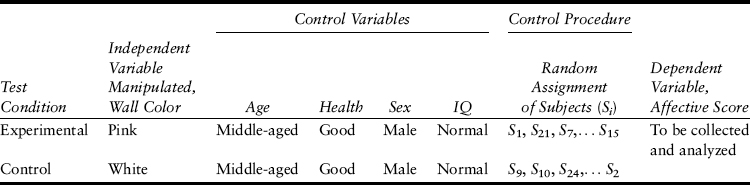With quantitative factors (e.g., dosage) as opposed to qualitative factors (e.g., type of drug), one may ascertain trends in the data when a factor has three or more levels (see Row b). Specifically, a minimum of three levels is required for ascertaining a linear trend, and a minimum of four levels for a quadratic trend.

### Table 2 Genres of Experimental Designs in Terms of Treatment Combinations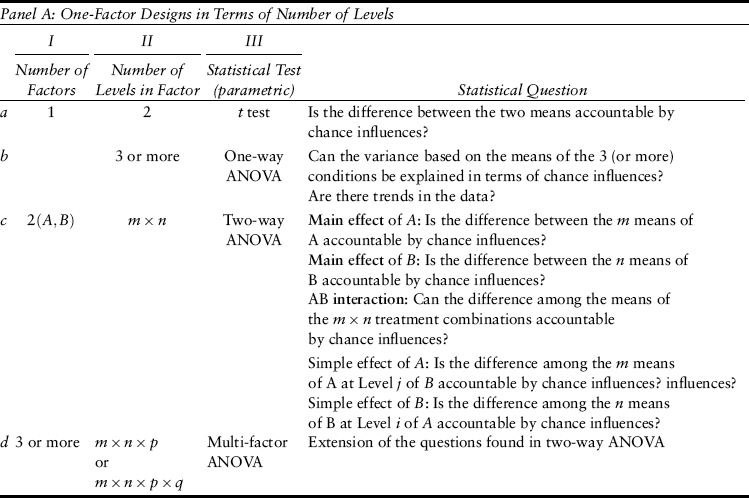### Two-Factor Designs

Suppose that Factors A (e.g., room color) and B (e.g., room size) are used together in an experiment. Factor A has m levels; its two levels are a1 and a2 when m = 2. If Factor B has n levels (and if n = 2), the two levels of B are b1 and b2. The experiment has a factorial design when every level of A is combined with every level of B to define a test condition or treatment combination. The size of the factorial design is m by n; it has m-by-n treatment combinations. This notation may be generalized to reflect factorial design of any size.

Specifically, the number of integers in the name of the design indicates the number of independent variables, whereas the identities of the integers stand for the respective number of levels. For example, the name of a three-factor design is m by n by p; the first independent variable has m levels, the second has n levels, and the third has p levels (see Row d of Table 2).

The lone statistical question of a one-factor, two-level design (see Row a of Table 2) is asked separately for Factors A and B in the case of the two-factor design (see [a] and [b] in Row c of Table 2). Either of them is a main effect (see [a] and [b] in Row c) so as to distinguish it from a simple effect (see Row c). This distinction may be illustrated with Table 3.

### Main Effect

Assume an equal number of subjects in all treatment combinations. The means of a1 and a2 are 4.5 and 2.5, respectively (see the “Mean of ai” column in either panel of Table 3). The main effect of A is 2 (i.e., 4.5–2.5). In the same vein, the means of b1 and b2 are 4 and 3, respectively (see the “Mean of bj” row in either panel of Table 3). The main effect of B is 1. That is, the two levels of B (or A) are averaged when the main effect of A (or B) is being considered.

### Table 3 What May Be Learned From a 2-by-2 Factorial Design (a)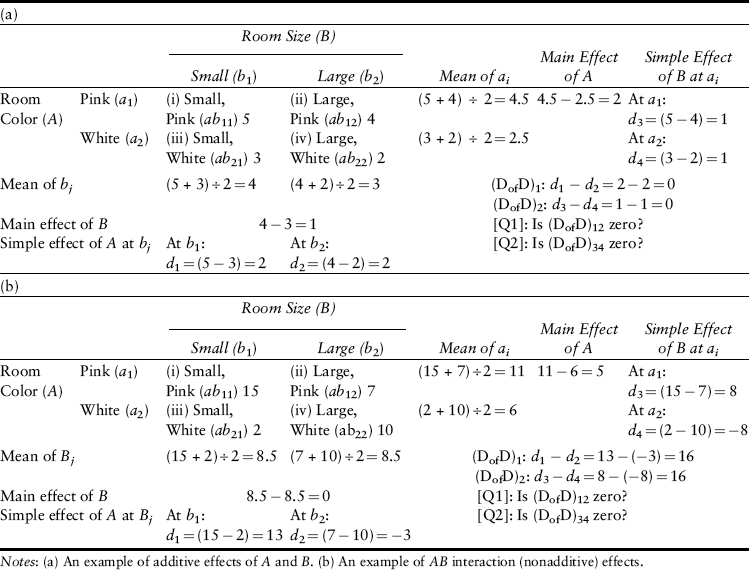### Simple Effect

Given that there are two levels of A (or B), it is possible to ask whether or not the two levels of B (or A) differ at either level of A (or B). Hence, there are the entries, d3 and d4, in the “Simple effect of B at ai” column, and the entries, d1 and d2, “Simple effect of A at bj” row in either panel of Table 3. Those entries are the four simple effects of the 2-by-2 factorial experiment. They may be summarized as follows: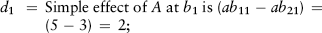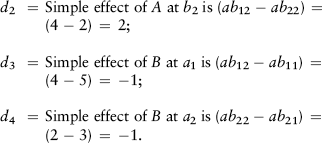### AB Interaction

In view of the fact that there are two simple effects of A (or B), it is important to know whether or not they differ. Consequently, the effects noted above give rise to the following questions: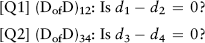Given that d1d2 = 0, one is informed that the effect of Variable A is independent of that of Variable B. By the same token, that d3d4 = 0 means that the effect of Variable B is independent of that of Variable A. That is to say, when the answers to both [Q1] and [Q2] are “Yes,” the joint effects of Variables A and B on the dependent variable are the sum of the individual effects of Variables A and B. Variables A and B are said to be additive in such an event.

Panel (b) of Table 3 illustrates a different scenario. The answers to both [Q1] and [Q2] are “No.” It informs one that the effects of Variable A (or B) on the dependent variable differ at different levels of Variable B (or A). In short, it is learned from a “No” answer to either [Q1] or [Q2] (or both) that the joint effects of Variables A and B on the dependent variables are nonadditive in the sense that their joint effects are not the simple sum of the two separate effects. Variables A and B are said to interact (or there is a two-way AB interaction) in such an event.

### Multifactor Designs

What has been said about two-factor designs also applies to designs with three or more independent variables (i.e., multifactor designs). For example, in the case of a three-factor design, it is possible to ask questions about three main effects (A, B, and C); three 2-way interaction effects (AB, AC, and BC interactions); a set of simple effects (e.g., the effect of Variable C at different treatment combinations of AB, etc.); and a three-way interaction (viz., ABC interaction).

### Genres of Experimental Designs for Data Interpretation Purposes

Experimental designs may also be classified in terms of how subjects are assigned to the treatment combinations, namely, completely randomized, repeated measures, randomized block, and split-plot.

### Completely Randomized Design

Suppose that there are 36 prospective subjects. As it is always advisable to assign an equal number of subjects to each treatment combination, six of them are assigned randomly to each of the six treatment combinations of a 2-by-3 factorial experiment. It is called the completely randomized design, but more commonly known as an unrelated sample (or an independent sample) design when there are only two levels to a lone independent variable.

### Repeated Measures Design

All subjects are tested in all treatment combinations in a repeated measures design. It is known by the more familiar name related samples or dependent samples design when there are only two levels to a lone independent variable. The related samples case may be used to illustrate one complication, namely, the potential artifact of the order of testing effect.

Suppose that all subjects are tested at Level I (or II) before being tested at Level II (or I). Whatever the outcome might be, it is not clear whether the result is due to an inherent difference between Levels I and II or to the proactive effects of the level used first on the performance at the subsequent level of the independent variable. For this reason, a procedure is used to balance the order of testing.

Specifically, subjects are randomly assigned to two subgroups. Group 1 is tested with one order (e.g., Level I before Level II), whereas Group 2 is tested with the other order (Level II before Level I). The more sophisticated Latin square arrangement is used to balance the order of test when there are three or more levels to the independent variable.

### Randomized Block Design

The nature of the levels used to represent an independent variable may preclude the use of the repeated measures design. Suppose that the two levels of therapeutic method are surgery and radiation. As either of these levels has irrevocable consequences, subjects cannot be used in both conditions. Pairs of subjects have to be selected, assigned, and tested in the following manner.

Prospective subjects are first screened in terms of a set of relevant variables (body weight, severity of symptoms, etc.). Pairs of subjects who are identical (or similar within acceptable limits) are formed. One member of each pair is assigned randomly to surgery, and the other member to radiation. This matched-pair procedure is extended to matched triplets (or groups of four subjects matched in terms of a set of criteria) if there are three (or four) levels to the independent variable. Each member of the triplets (or four-member groups) is assigned randomly to one of the treatment combinations.

### Table 4 Inductive Principles Beyond the Method of Difference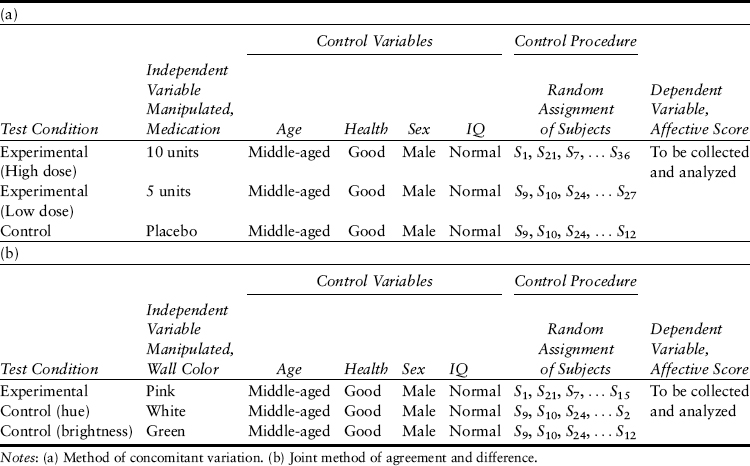### Split-Plot Design

A split-plot design is a combination of the repeated measures design and the completely randomized design. It is used when the levels of one of the independent variables has irrevocable effects (e.g., surgery or radiation of therapeutic method), whereas the other independent variable does not (e.g., Drugs A and B of type of drug).

### Underlying Inductive Logic

Designs other than the one-factor, two-level design implicate two other rules of induction, namely, the method of concomitant variation and the joint method of agreement and difference.

### Method of Concomitant Variation

Consider a study of the effects of a drug's dosage. The independent variable is dosage, whose three levels are 10, 5, and 0 units of the medication in question. As dosage is a quantitative variable, it is possible to ask whether or not the effect of treatment varies systematically with dosage. The experimental conditions are arranged in the way shown in Panel (a) of Table 4 that depicts the method of concomitant variation.

The control variables and procedures in Tables 1 and 4 are the same. The only difference is that each row in Table 4 represents a level (of a single independent variable) or a treatment combination (when there are two or more independent variables). That is to say, the method of concomitant variation is the logic underlying factorial designs of any size when quantitative independent variables are used.

### Joint Method of Agreement and Difference

Shown in Panel (b) of Table 4 is the joint method of agreement and disagreement. Whatever is true of Panel (a) of Table 4 also applies to Panel (b) of Table 4. It is the underlying inductive rule when a qualitative independent variable is used (e.g., room color).

In short, an experimental design is a stipulation of the formal arrangement of the independent, control, and independent variables, as well as the control procedure, of an experiment. Underlying every experimental design is an inductive rule that reduces ambiguity by rendering it possible to exclude alternative interpretations of the result. Each control variable or control procedure excludes one alternative explanation of the data.

Boring, E. G.The nature and history of experimental control.American Journal of Psychology,(1954).67,573–589.
(1992).Research methods in psychology: A primer.Calgary, Alberta, Canada: Detselig.
(1973).A system of logic: Ratiocinative and inductive.Toronto, Ontario, Canada: University of Toronto Press.
•••